Volume of a cuboid

## Objective

To obtain a formula for the volume of a cuboid.

## What is Cube and Cuboid?

Cube and cuboid shapes are three-dimensional shapes in mathematics. The cube and cuboid are formed by rotating the two-dimensional shapes square and rectangle, respectively.

Cube: A cube is a three-dimensional shape. It has six faces, eight vertices, and twelve edges. The cube's faces are all square and have equal dimensions.

Cuboid: A cuboid is a three-dimensional shape with six faces, eight vertices, and twelve edges. The cuboid has parallel faces. However, not all of a cuboid's faces are the same size.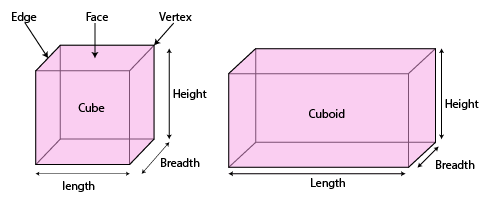Fig. (1) Cube and cuboid

## What is volume?

• If an object is solid, the space it occupies is measured and referred to as the volume of the object.
• If, on the other hand, the object is hollow, its interior is vacant and can be filled with air or any liquid that will take the shape of its container. In this case, the volume of the substance that may occupy the interior is referred to as the capacity of the container.
• In short, an object's volume is the amount of space it takes up, and its capacity is the amount of substance it can hold in its interior.
• Hence, the unit of measurement for either of the two is a cubic unit.
• The length, breadth, and height of a cuboid determine its volume; hence, altering any one of those dimensions will change the volume of the shape.

## Volume of Cuboid

• The formula for cuboid volume can be obtained from the concept of rectangular sheets.
• Let the area of a rectangular sheet of paper be 'A', the volume of the cuboid be 'V' , and the height up to which they are stacked be 'h'.
• Can you tell what the relationship between V, A, and h would be?
• The area of the plane region occupied by each rectangle multiplied by its height equals the volume of the cuboid.
• As a result, A x h = V
• That is,
• A cuboid's volume is determined by multiplying the base area by the height.
• The base area of a cuboid is equal to l x b.
• Therefore, V = l x b x h = lbh is the formula for a cuboid's volume.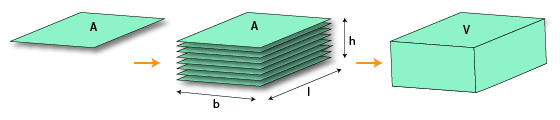Fig. (2) Volume of cuboid

for example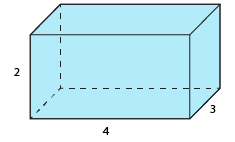Fig. (3) Cuboid

Let a cuboid's length be 4 units, its breadth be 3 units, and its height be 2 units, as shown in Fig. (3).
Then, by the formula, the volume of the cuboid is equal to (length x breadth x height) cubic units.
By putting the values in the formula, we get the volume as 24 cubic units.

## Prerequisite knowledge

Students should already be familiar with

• Rules of multiplication
• Area of a rectangle and a square

Concept of area in a rectangle:

•  Let us consider a rectangle ABCD of length l units and breadth b units.
• Then, the area of the rectangle ABCD can be calculated as l*b unit².
•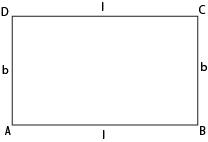Fig. (4) Rectangle

Concept of Area of square:

Let us say a square ABCD of
Side = x
Then, Area of square ABCD can be defined as= x*x= x²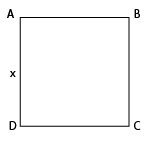Fig. (5) Square